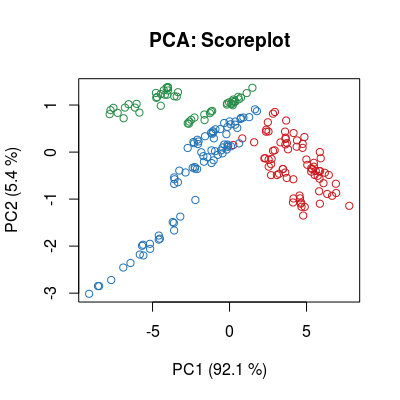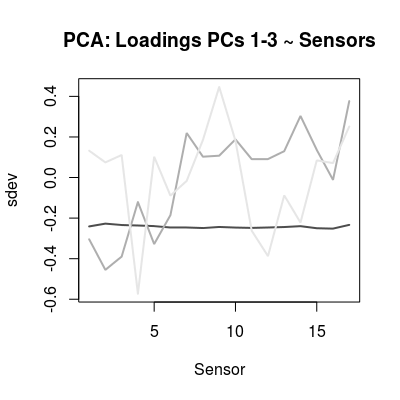Dataset UNIMANshort.

Description

Short-term UNIMAN datasets of 200 samples from 17 polymeric sensors. The datasets contains two matricies:

 C The concentration matrix of 200 rows and 3 columns encodes the concentration profile for three gases, ammonia, propanoic acid and n-buthanol. The concentration units are given in the percentage volume (% vol.). Ammonia has three concentration levels 0.01, 0.02 and 0.05, propanoic acid - three levels 0.01, 0.02 and 0.05, and n-buthanol - two levels 0.1 and 1. dat The data matrix of 200 rows and 17 columns cotains the steady-state signals of 17 sensors in response to the concentration profle C.

Details

The reference dataset has been measured at The University of Manchester (UNIMAN). Three analytes ammonia, propanoic acid and n-buthanol, at different concentration levels, were measured for 10 months with an array of seventeen conducting polymer sensors.

In modeling of the array we make the distinction between short-term and long-term reference data. Two hundred samples from the first 6 days are used to characterize the array assuming the absence of drift. The long-term reference data (not published within the package) counts for the complete number of samples from 10 months, these data were used to model the sensor noise and drift, see UNIMANsnoise and UNIMANdnoise for more details.

A pre-processing procedure on outliers removal was applied to the reference data. The standard method based on the squared Mahalanobis distance was used with quantile equal to 0.975%.

Examples

data("UNIMANshort", package="chemosensors") str(UNIMANshort)
List of 3 \$ C : num [1:200, 1:3] 0.01 0.01 0.01 0.01 0.01 0.02 0.02 0.02 0.02 0.02 ... ..- attr(*, "dimnames")=List of 2 .. ..\$ : NULL .. ..\$ : chr [1:3] "Ammonia" "Propanoic" "n-buthanol" \$ dat : num [1:200, 1:17] 8.9 8.88 8.87 8.86 8.73 ... \$ dat.corrected: num [1:200, 1:17] 8.56 8.6 8.53 8.58 8.53 ... ..- attr(*, "scaled:center")= num [1:17] 8.53 8.31 8.08 5.99 7.77 ... ..- attr(*, "scaled:scale")= num [1:17] 0.729 0.544 0.655 0.387 0.684 ...
C <- UNIMANshort\$C dat <- UNIMANshort\$dat # plot sensors in affinity space of gases #plotAffinitySpace(conc=C, sdata=dat, gases=c(2, 1)) #plotAffinitySpace(conc=C, sdata=dat, gases=c(2, 3)) #plotAffinitySpace(conc=C, sdata=dat, gases=c(3, 1)) # make standar PCA (package 'pls') to see: # - multi-variate class distribution (scoreplot) # - low-dimensionality of data (variance) # - contribution of 17 sensors in terms of linear modeling (loadings) mod <- prcomp(dat, center=TRUE, scale=TRUE) col <- ccol(C) scoreplot(mod, col=col, main="PCA: Scoreplot")barplot(mod\$sdev, main="PCA: Sd. Deviation ~ PCs")